Intermediate Algebra

# 10.1Finding Composite and Inverse Functions

Intermediate Algebra10.1 Finding Composite and Inverse Functions

### Learning Objectives

By the end of this section, you will be able to:
• Find and evaluate composite functions
• Determine whether a function is one-to-one
• Find the inverse of a function

### Be Prepared 10.1

Before you get started, take this readiness quiz.

1. If $f(x)=2x−3f(x)=2x−3$ and $g(x)=x2+2x−3,g(x)=x2+2x−3,$ find $f(4).f(4).$
If you missed this problem, review Example 3.48.
2. Solve for $x,x,$ $3x+2y=12.3x+2y=12.$
If you missed this problem, review Example 2.31.
3. Simplify: $5(x+4)5−4.5(x+4)5−4.$
If you missed this problem, review Example 1.25.

In this chapter, we will introduce two new types of functions, exponential functions and logarithmic functions. These functions are used extensively in business and the sciences as we will see.

### Find and Evaluate Composite Functions

Before we introduce the functions, we need to look at another operation on functions called composition. In composition, the output of one function is the input of a second function. For functions $ff$ and $g,g,$ the composition is written $f∘gf∘g$ and is defined by $(f∘g)(x)=f(g(x)).(f∘g)(x)=f(g(x)).$

We read $f(g(x))f(g(x))$ as $“f“f$ of $gg$ of $x.”x.”$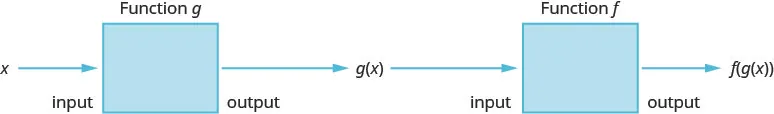To do a composition, the output of the first function, $g(x),g(x),$ becomes the input of the second function, f, and so we must be sure that it is part of the domain of f.

### Composition of Functions

The composition of functions f and g is written $f·gf·g$ and is defined by

$(f∘g)(x)=f(g(x))(f∘g)(x)=f(g(x))$

We read $f(g(x))f(g(x))$ as $ff$ of $gg$ of x.

We have actually used composition without using the notation many times before. When we graphed quadratic functions using translations, we were composing functions. For example, if we first graphed $g(x)=x2g(x)=x2$ as a parabola and then shifted it down vertically four units, we were using the composition defined by $(f∘g)(x)=f(g(x))(f∘g)(x)=f(g(x))$ where $f(x)=x−4.f(x)=x−4.$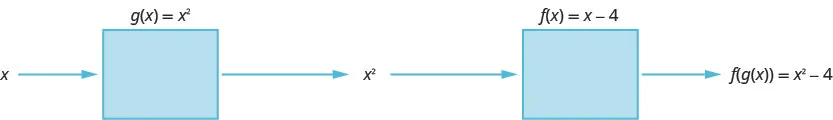The next example will demonstrate that $(f∘g)(x),(f∘g)(x),$ $(g∘f)(x)(g∘f)(x)$ and $(f·g)(x)(f·g)(x)$ usually result in different outputs.

### Example 10.1

For functions $f(x)=4x−5f(x)=4x−5$ and $g(x)=2x+3,g(x)=2x+3,$ find: $(f∘g)(x),(f∘g)(x),$ $(g∘f)(x),(g∘f)(x),$ and $(f·g)(x).(f·g)(x).$

### Try It 10.1

For functions $f(x)=3x−2f(x)=3x−2$ and $g(x)=5x+1,g(x)=5x+1,$ find $(f∘g)(x)(f∘g)(x)$ $(g∘f)(x)(g∘f)(x)$ $(f·g)(x)(f·g)(x)$.

### Try It 10.2

For functions $f(x)=4x−3,f(x)=4x−3,$ and $g(x)=6x−5,g(x)=6x−5,$ find $(f∘g)(x),(f∘g)(x),$ $(g∘f)(x),(g∘f)(x),$ and $(f·g)(x).(f·g)(x).$

In the next example we will evaluate a composition for a specific value.

### Example 10.2

For functions $f(x)=x2−4,f(x)=x2−4,$ and $g(x)=3x+2,g(x)=3x+2,$ find: $(f∘g)(−3),(f∘g)(−3),$ $(g∘f)(−1),(g∘f)(−1),$ and $(f∘f)(2).(f∘f)(2).$

### Try It 10.3

For functions $f(x)=x2−9,f(x)=x2−9,$ and $g(x)=2x+5,g(x)=2x+5,$ find $(f∘g)(−2),(f∘g)(−2),$ $(g∘f)(−3),(g∘f)(−3),$ and $(f∘f)(4).(f∘f)(4).$

### Try It 10.4

For functions $f(x)=x2+1,f(x)=x2+1,$ and $g(x)=3x−5,g(x)=3x−5,$ find $(f∘g)(−1),(f∘g)(−1),$ $(g∘f)(2),(g∘f)(2),$ and $(f∘f)(−1).(f∘f)(−1).$

### Determine Whether a Function is One-to-One

When we first introduced functions, we said a function is a relation that assigns to each element in its domain exactly one element in the range. For each ordered pair in the relation, each x-value is matched with only one y-value.

We used the birthday example to help us understand the definition. Every person has a birthday, but no one has two birthdays and it is okay for two people to share a birthday. Since each person has exactly one birthday, that relation is a function.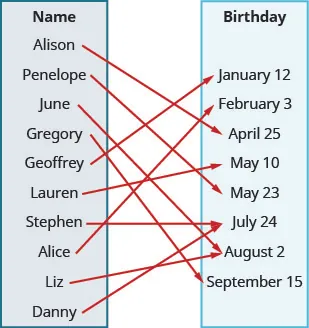A function is one-to-one if each value in the range has exactly one element in the domain. For each ordered pair in the function, each y-value is matched with only one x-value.

Our example of the birthday relation is not a one-to-one function. Two people can share the same birthday. The range value August 2 is the birthday of Liz and June, and so one range value has two domain values. Therefore, the function is not one-to-one.

### One-to-One Function

A function is one-to-one if each value in the range corresponds to one element in the domain. For each ordered pair in the function, each y-value is matched with only one x-value. There are no repeated y-values.

### Example 10.3

For each set of ordered pairs, determine if it represents a function and, if so, if the function is one-to-one.

${(−3,27),(−2,8),(−1,1),(0,0),(1,1),(2,8),(3,27)}{(−3,27),(−2,8),(−1,1),(0,0),(1,1),(2,8),(3,27)}$ and ${(0,0),(1,1),(4,2),(9,3),(16,4)}.{(0,0),(1,1),(4,2),(9,3),(16,4)}.$

### Try It 10.5

For each set of ordered pairs, determine if it represents a function and if so, is the function one-to-one.

${(−3,−6),(−2,−4),(−1,−2),(0,0),(1,2),(2,4),(3,6)}{(−3,−6),(−2,−4),(−1,−2),(0,0),(1,2),(2,4),(3,6)}$ ${(−4,8),(−2,4),(−1,2),(0,0),(1,2),(2,4),(4,8)}{(−4,8),(−2,4),(−1,2),(0,0),(1,2),(2,4),(4,8)}$

### Try It 10.6

For each set of ordered pairs, determine if it represents a function and if so, is the function one-to-one.

${(27,−3),(8,−2),(1,−1),(0,0),(1,1),(8,2),(27,3)}{(27,−3),(8,−2),(1,−1),(0,0),(1,1),(8,2),(27,3)}$ ${(7,−3),(−5,−4),(8,0),(0,0),(−6,4),(−2,2),(−1,3)}{(7,−3),(−5,−4),(8,0),(0,0),(−6,4),(−2,2),(−1,3)}$

To help us determine whether a relation is a function, we use the vertical line test. A set of points in a rectangular coordinate system is the graph of a function if every vertical line intersects the graph in at most one point. Also, if any vertical line intersects the graph in more than one point, the graph does not represent a function.

The vertical line is representing an x-value and we check that it intersects the graph in only one y-value. Then it is a function.

To check if a function is one-to-one, we use a similar process. We use a horizontal line and check that each horizontal line intersects the graph in only one point. The horizontal line is representing a y-value and we check that it intersects the graph in only one x-value. If every horizontal line intersects the graph of a function in at most one point, it is a one-to-one function. This is the horizontal line test.

### Horizontal Line Test

If every horizontal line intersects the graph of a function in at most one point, it is a one-to-one function.

We can test whether a graph of a relation is a function by using the vertical line test. We can then tell if the function is one-to-one by applying the horizontal line test.

### Example 10.4

Determine whether each graph is the graph of a function and, if so, whether it is one-to-one.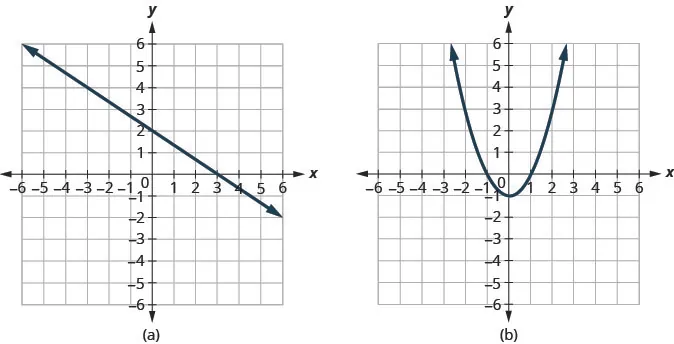### Try It 10.7

Determine whether each graph is the graph of a function and, if so, whether it is one-to-one.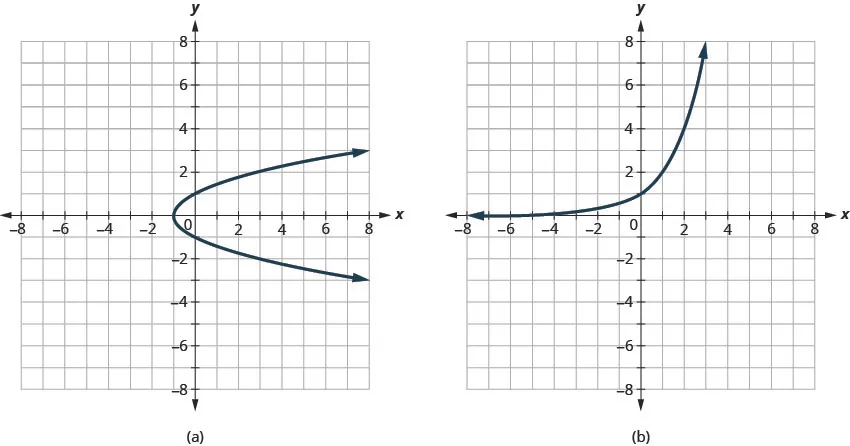### Try It 10.8

Determine whether each graph is the graph of a function and, if so, whether it is one-to-one.### Find the Inverse of a Function

Let’s look at a one-to one function, $ff$, represented by the ordered pairs ${(0,5),(1,6),(2,7),(3,8)}.{(0,5),(1,6),(2,7),(3,8)}.$ For each $xx$-value, $ff$ adds 5 to get the $yy$-value. To ‘undo’ the addition of 5, we subtract 5 from each $yy$-value and get back to the original $xx$-value. We can call this “taking the inverse of $ff$” and name the function $f−1.f−1.$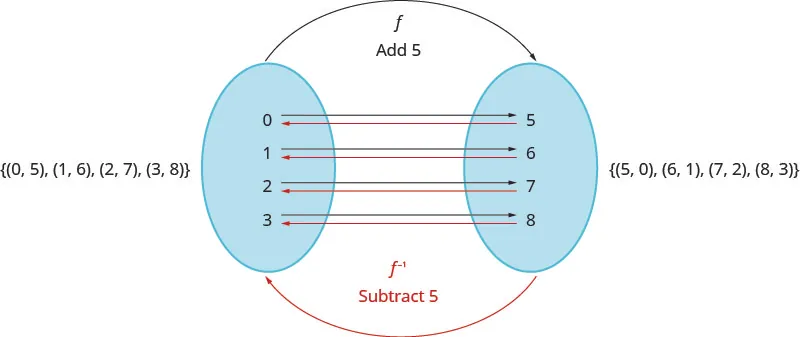Notice that that the ordered pairs of $ff$ and $f−1f−1$ have their $xx$-values and $yy$-values reversed. The domain of $ff$ is the range of $f−1f−1$ and the domain of $f−1f−1$ is the range of $f.f.$

### Inverse of a Function Defined by Ordered Pairs

If $f(x)f(x)$ is a one-to-one function whose ordered pairs are of the form $(x,y),(x,y),$ then its inverse function $f−1(x)f−1(x)$ is the set of ordered pairs $(y,x).(y,x).$

In the next example we will find the inverse of a function defined by ordered pairs.

### Example 10.5

Find the inverse of the function ${(0,3),(1,5),(2,7),(3,9)}.{(0,3),(1,5),(2,7),(3,9)}.$ Determine the domain and range of the inverse function.

### Try It 10.9

Find the inverse of ${(0,4),(1,7),(2,10),(3,13)}.{(0,4),(1,7),(2,10),(3,13)}.$ Determine the domain and range of the inverse function.

### Try It 10.10

Find the inverse of ${(−1,4),(−2,1),(−3,0),(−4,2)}.{(−1,4),(−2,1),(−3,0),(−4,2)}.$ Determine the domain and range of the inverse function.

We just noted that if $f(x)f(x)$ is a one-to-one function whose ordered pairs are of the form $(x,y),(x,y),$ then its inverse function $f−1(x)f−1(x)$ is the set of ordered pairs $(y,x).(y,x).$

So if a point $(a,b)(a,b)$ is on the graph of a function $f(x),f(x),$ then the ordered pair $(b,a)(b,a)$ is on the graph of $f−1(x).f−1(x).$ See Figure 10.2.

Figure 10.2

The distance between any two pairs $(a,b)(a,b)$ and $(b,a)(b,a)$ is cut in half by the line $y=x.y=x.$ So we say the points are mirror images of each other through the line $y=x.y=x.$

Since every point on the graph of a function $f(x)f(x)$ is a mirror image of a point on the graph of $f−1(x),f−1(x),$ we say the graphs are mirror images of each other through the line $y=x.y=x.$ We will use this concept to graph the inverse of a function in the next example.

### Example 10.6

Graph, on the same coordinate system, the inverse of the one-to one function shown.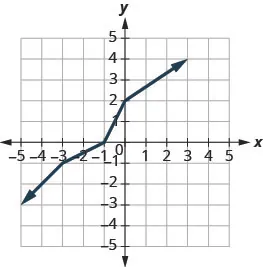### Try It 10.11

Graph, on the same coordinate system, the inverse of the one-to one function.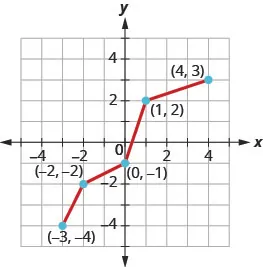### Try It 10.12

Graph, on the same coordinate system, the inverse of the one-to one function.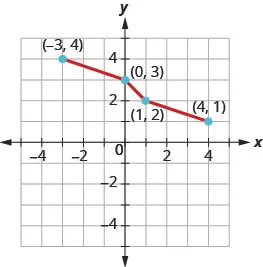When we began our discussion of an inverse function, we talked about how the inverse function ‘undoes’ what the original function did to a value in its domain in order to get back to the original x-value.### Inverse Functions

$f−1(f(x))=x,for allxin the domain offf(f−1(x))=x,for allxin the domain off−1f−1(f(x))=x,for allxin the domain offf(f−1(x))=x,for allxin the domain off−1$

We can use this property to verify that two functions are inverses of each other.

### Example 10.7

Verify that $f(x)=5x−1f(x)=5x−1$ and $g(x)=x+15g(x)=x+15$ are inverse functions.

### Try It 10.13

Verify that the functions are inverse functions.

$f(x)=4x−3f(x)=4x−3$ and $g(x)=x+34.g(x)=x+34.$

### Try It 10.14

Verify that the functions are inverse functions.

$f(x)=2x+6f(x)=2x+6$ and $g(x)=x−62.g(x)=x−62.$

We have found inverses of function defined by ordered pairs and from a graph. We will now look at how to find an inverse using an algebraic equation. The method uses the idea that if $f(x)f(x)$ is a one-to-one function with ordered pairs $(x,y),(x,y),$ then its inverse function $f−1(x)f−1(x)$ is the set of ordered pairs $(y,x).(y,x).$

If we reverse the x and y in the function and then solve for y, we get our inverse function.

### Example 10.8

#### How to Find the inverse of a One-to-One Function

Find the inverse of $f(x)=4x+7.f(x)=4x+7.$

### Try It 10.15

Find the inverse of the function $f(x)=5x−3.f(x)=5x−3.$

### Try It 10.16

Find the inverse of the function $f(x)=8x+5.f(x)=8x+5.$

We summarize the steps below.

### How To

#### How to Find the inverse of a One-to-One Function

1. Step 1. Substitute y for $f(x).f(x).$
2. Step 2. Interchange the variables x and y.
3. Step 3. Solve for y.
4. Step 4. Substitute $f−1(x)f−1(x)$ for y.
5. Step 5. Verify that the functions are inverses.

### Example 10.9

#### How to Find the Inverse of a One-to-One Function

Find the inverse of $f(x)=2x−35.f(x)=2x−35.$

### Try It 10.17

Find the inverse of the function $f(x)=3x−25.f(x)=3x−25.$

### Try It 10.18

Find the inverse of the function $f(x)=6x−74.f(x)=6x−74.$

### Section 10.1 Exercises

#### Practice Makes Perfect

Find and Evaluate Composite Functions

In the following exercises, find (fg)(x), (gf)(x), and (f · g)(x).

1.

$f(x)=4x+3f(x)=4x+3$ and $g(x)=2x+5g(x)=2x+5$

2.

$f(x)=3x−1f(x)=3x−1$ and $g(x)=5x−3g(x)=5x−3$

3.

$f(x)=6x−5f(x)=6x−5$ and $g(x)=4x+1g(x)=4x+1$

4.

$f(x)=2x+7f(x)=2x+7$ and $g(x)=3x−4g(x)=3x−4$

5.

$f(x)=3xf(x)=3x$ and $g(x)=2x2−3xg(x)=2x2−3x$

6.

$f(x)=2xf(x)=2x$ and $g(x)=3x2−1g(x)=3x2−1$

7.

$f(x)=2x−1f(x)=2x−1$ and $g(x)=x2+2g(x)=x2+2$

8.

$f(x)=4x+3f(x)=4x+3$ and $g(x)=x2−4g(x)=x2−4$

In the following exercises, find the values described.

9.

For functions $f(x)=2x2+3f(x)=2x2+3$ and $g(x)=5x−1,g(x)=5x−1,$ find $(f∘g)(−2)(f∘g)(−2)$ $(g∘f)(−3)(g∘f)(−3)$ $(f∘f)(−1)(f∘f)(−1)$

10.

For functions $f(x)=5x2−1f(x)=5x2−1$ and $g(x)=4x−1,g(x)=4x−1,$ find $(f∘g)(1)(f∘g)(1)$ $(g∘f)(−1)(g∘f)(−1)$ $(f∘f)(2)(f∘f)(2)$

11.

For functions $f(x)=2x3f(x)=2x3$ and $g(x)=3x2+2,g(x)=3x2+2,$ find $(f∘g)(−1)(f∘g)(−1)$ $(g∘f)(1)(g∘f)(1)$ $(g∘g)(1)(g∘g)(1)$

12.

For functions $f(x)=3x3+1f(x)=3x3+1$ and $g(x)=2x2−3,g(x)=2x2−3,$ find $(f∘g)(−2)(f∘g)(−2)$ $(g∘f)(−1)(g∘f)(−1)$ $(g∘g)(1)(g∘g)(1)$

Determine Whether a Function is One-to-One

In the following exercises, determine if the set of ordered pairs represents a function and if so, is the function one-to-one.

13.

${(−3,9),(−2,4),(−1,1),(0,0){(−3,9),(−2,4),(−1,1),(0,0)$,
$(1,1),(2,4),(3,9)}(1,1),(2,4),(3,9)}$

14.

${(9,−3),(4,−2),(1,−1),(0,0){(9,−3),(4,−2),(1,−1),(0,0)$,
$(1,1),(4,2),(9,3)}(1,1),(4,2),(9,3)}$

15.

${(−3,−5),(−2,−3),(−1,−1){(−3,−5),(−2,−3),(−1,−1)$,
$(0,1),(1,3),(2,5),(3,7)}(0,1),(1,3),(2,5),(3,7)}$

16.

${(5,3),(4,2),(3,1),(2,0){(5,3),(4,2),(3,1),(2,0)$,
$(1,−1),(0,−2),(−1,−3)}(1,−1),(0,−2),(−1,−3)}$

In the following exercises, determine whether each graph is the graph of a function and if so, is it one-to-one.

17.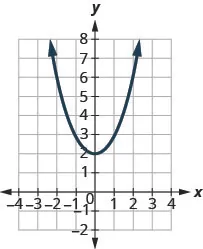18.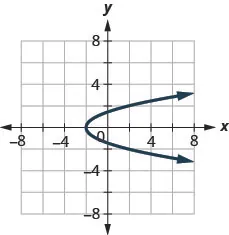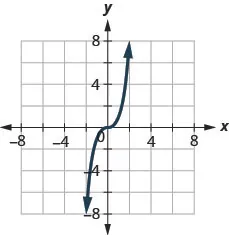19.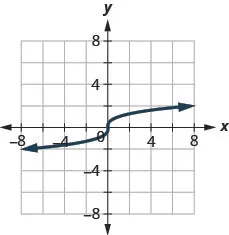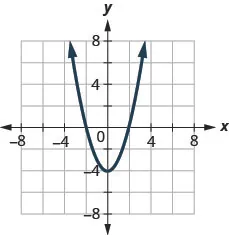20.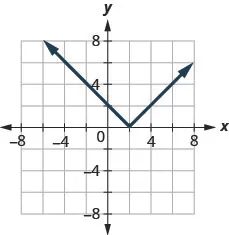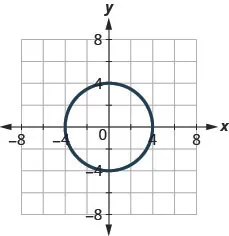In the following exercises, find the inverse of each function. Determine the domain and range of the inverse function.

21.

${ ( 2 , 1 ) , ( 4 , 2 ) , ( 6 , 3 ) , ( 8 , 4 ) } { ( 2 , 1 ) , ( 4 , 2 ) , ( 6 , 3 ) , ( 8 , 4 ) }$

22.

${ ( 6 , 2 ) , ( 9 , 5 ) , ( 12 , 8 ) , ( 15 , 11 ) } { ( 6 , 2 ) , ( 9 , 5 ) , ( 12 , 8 ) , ( 15 , 11 ) }$

23.

${ ( 0 , −2 ) , ( 1 , 3 ) , ( 2 , 7 ) , ( 3 , 12 ) } { ( 0 , −2 ) , ( 1 , 3 ) , ( 2 , 7 ) , ( 3 , 12 ) }$

24.

${ ( 0 , 0 ) , ( 1 , 1 ) , ( 2 , 4 ) , ( 3 , 9 ) } { ( 0 , 0 ) , ( 1 , 1 ) , ( 2 , 4 ) , ( 3 , 9 ) }$

25.

${ ( −2 , −3 ) , ( −1 , −1 ) , ( 0 , 1 ) , ( 1 , 3 ) } { ( −2 , −3 ) , ( −1 , −1 ) , ( 0 , 1 ) , ( 1 , 3 ) }$

26.

${ ( 5 , 3 ) , ( 4 , 2 ) , ( 3 , 1 ) , ( 2 , 0 ) } { ( 5 , 3 ) , ( 4 , 2 ) , ( 3 , 1 ) , ( 2 , 0 ) }$

In the following exercises, graph, on the same coordinate system, the inverse of the one-to-one function shown.

27.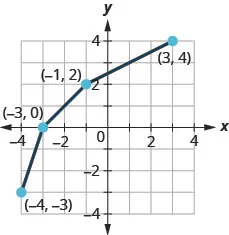28.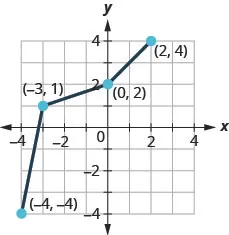29.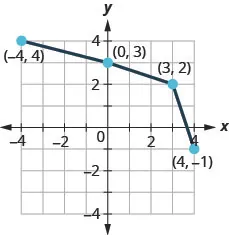30.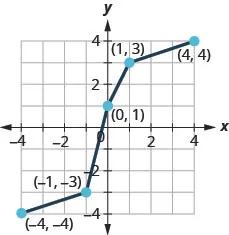In the following exercises, determine whether or not the given functions are inverses.

31.

$f(x)=x+8f(x)=x+8$ and $g(x)=x−8g(x)=x−8$

32.

$f(x)=x−9f(x)=x−9$ and $g(x)=x+9g(x)=x+9$

33.

$f(x)=7xf(x)=7x$ and $g(x)=x7g(x)=x7$

34.

$f(x)=x11f(x)=x11$ and $g(x)=11xg(x)=11x$

35.

$f(x)=7x+3f(x)=7x+3$ and $g(x)=x−37g(x)=x−37$

36.

$f(x)=5x−4f(x)=5x−4$ and $g(x)=x−45g(x)=x−45$

37.

$f(x)=x+2f(x)=x+2$ and $g(x)=x2−2g(x)=x2−2$

38.

$f(x)=x−43f(x)=x−43$ and $g(x)=x3+4g(x)=x3+4$

In the following exercises, find the inverse of each function.

39.

$f ( x ) = x − 12 f ( x ) = x − 12$

40.

$f ( x ) = x + 17 f ( x ) = x + 17$

41.

$f ( x ) = 9 x f ( x ) = 9 x$

42.

$f ( x ) = 8 x f ( x ) = 8 x$

43.

$f ( x ) = x 6 f ( x ) = x 6$

44.

$f ( x ) = x 4 f ( x ) = x 4$

45.

$f ( x ) = 6 x − 7 f ( x ) = 6 x − 7$

46.

$f ( x ) = 7 x − 1 f ( x ) = 7 x − 1$

47.

$f ( x ) = −2 x + 5 f ( x ) = −2 x + 5$

48.

$f ( x ) = −5 x − 4 f ( x ) = −5 x − 4$

49.

$f(x)=x2+6,f(x)=x2+6,$ $x≥0x≥0$

50.

$f(x)=x2−9,f(x)=x2−9,$ $x≥0x≥0$

51.

$f ( x ) = x 3 − 4 f ( x ) = x 3 − 4$

52.

$f ( x ) = x 3 + 6 f ( x ) = x 3 + 6$

53.

$f ( x ) = 1 x + 2 f ( x ) = 1 x + 2$

54.

$f ( x ) = 1 x − 6 f ( x ) = 1 x − 6$

55.

$f(x)=x−2,f(x)=x−2,$ $x≥2x≥2$

56.

$f(x)=x+8,f(x)=x+8,$ $x≥−8x≥−8$

57.

$f ( x ) = x − 3 3 f ( x ) = x − 3 3$

58.

$f ( x ) = x + 5 3 f ( x ) = x + 5 3$

59.

$f(x)=9x−54,f(x)=9x−54,$ $x≥59x≥59$

60.

$f(x)=8x−34,f(x)=8x−34,$ $x≥38x≥38$

61.

$f ( x ) = −3 x + 5 5 f ( x ) = −3 x + 5 5$

62.

$f ( x ) = −4 x − 3 5 f ( x ) = −4 x − 3 5$

#### Writing Exercises

63.

Explain how the graph of the inverse of a function is related to the graph of the function.

64.

Explain how to find the inverse of a function from its equation. Use an example to demonstrate the steps.

#### Self Check

After completing the exercises, use this checklist to evaluate your mastery of the objectives of this section.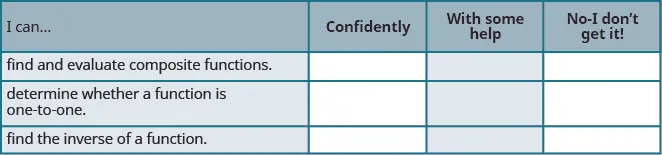If most of your checks were:

…confidently. Congratulations! You have achieved the objectives in this section. Reflect on the study skills you used so that you can continue to use them. What did you do to become confident of your ability to do these things? Be specific.

…with some help. This must be addressed quickly because topics you do not master become potholes in your road to success. In math every topic builds upon previous work. It is important to make sure you have a strong foundation before you move on. Who can you ask for help? Your fellow classmates and instructor are good resources. Is there a place on campus where math tutors are available? Can your study skills be improved?

…no—I don’t get it! This is a warning sign and you must not ignore it. You should get help right away or you will quickly be overwhelmed. See your instructor as soon as you can to discuss your situation. Together you can come up with a plan to get you the help you need.

Order a print copy

As an Amazon Associate we earn from qualifying purchases.